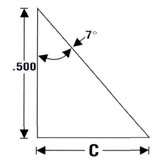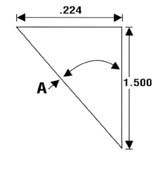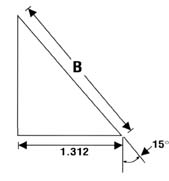# Trigonometry

By Staff
1 / 32 / 33 / 3Perhaps some of my fellow shade-tree machinists would have a use
for my easy way to figure the three most common angles.

First you need a scientific calculator, such as a Texas
Instruments TI-30, which costs approximately \$11. Then you
substitute your known numbers and angles for the ones in the three
problems (see examples). Keep in mind that with angles, the numbers
represent degrees, minutes and seconds, in that order.

To Find A

Use the numbers to the left of the decimals point for your
angle.
8.
49
(Degrees)
29.4
(Minutes)
24.0
(Seconds)

Use the numbers to the right of the decimal point to figure the
problem.
8.49
29.4
24.0

To Find A
Punch 0.224
Punch
Punch 1.500
Punch = 0.149
Punch INV
Punch TAN = 8.49
Punch 0.49
Punch x
Punch 60
Punch = 29.4
Punch 0.4
Punch x
Punch 60
Punch = 24
A = 8°, 29°, 24′

To Find B and C

Follow my format and you should have no trouble.

The tricky part of B and C is if your angle is 15°22′.

Let’s do B
Divide 22 into 60
Punch 22
Punch
Punch 60
Punch =

To Find B
Punch 1.312
Punch
Punch 15
Punch SIN
Punch = 5.069

B = 5.069

To Find C
Punch .500
Punch x
Punch 7
Punch TAN
Punch = 0.061

C = 0.061